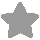Informatica Online Judge

##Factorials (Eng) [0295 / 0127]Time Limit(Test case) : 1000(ms)
Number of users who solved : 81   Total Tried : 523

The Champion of this Problem (C++) : tlsdydaud1 - 0ms / 112byte
My Best Submission (C++) : N/A

[]

#### Background

The factorial of an integer N, written N!, is the product of all the integers from 1 through N inclusive.

The factorial quickly becomes very large: 13! is too large to store in a 32-bit integer on most computers, and 70! is too large for most floating-point variables.

For example, 5! = 1 * 2 * 3 * 4 * 5 = 120, so the rightmost non-zero digit of 5! is 2. Likewise, 7! = 1 * 2 * 3 * 4 * 5 * 6 * 7 = 5040, so the rightmost non-zero digit of 7! is 4.

#### Input

A single positive integer N no larger than 4,220.

#### Output

A single line containing but a single digit: the right most non-zero digit of N! .

#### IO Example

Input
7

Output
4

Submit : [C/C++] | [C++11] | [Obj-C] | [Java] | [Python]
Prob Analysis : [Problem Statistics] | [Solution]